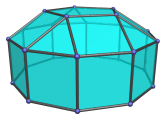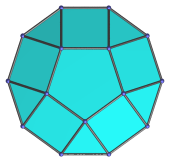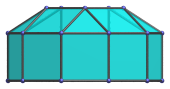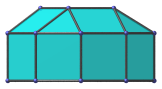# The Elongated Pentagonal Cupola

The elongated pentagonal cupola is the 20th Johnson solid (J20). It has 25 vertices, 45 edges, and 22 faces (5 equilateral triangles, 15 squares, 1 pentagon, and 1 decagon).The elongated pentagonal cupola can be constructed by attaching a decagonal prism to a pentagonal cupola (J5), lengthening it; hence the name elongated.

## Projections

Here are some views of the elongated pentagonal cupola from various angles:

Projection Envelope DescriptionRegular decagon

Top view.Hexagon

Front view.Hexagon

Side view.

## Coordinates

The Cartesian coordinates of the elongated pentagonal cupola with edge length 2 are:

• (0, √((10+2√(5))/5), 1+2√((3-φ)/5))
• (±φ, √((5-√(5))/10), 1+2√((3-φ)/5))
• (±1, -√((5+2√(5))/5), 1+2√((3-φ)/5))
• (±2φ, 0, ±1)
• (±1, ±√(3+4φ), ±1)
• (±φ2, ±√(2+φ), ±1)

where φ=(1+√5)/2 is the Golden Ratio.

Last updated 18 Jun 2019.